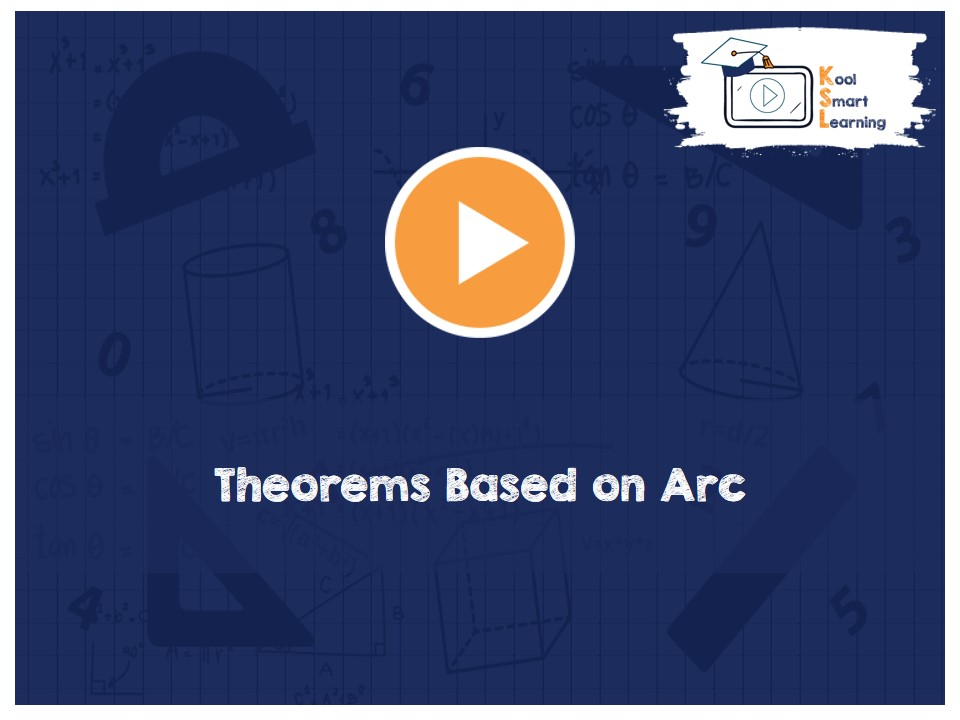At KoolSmartLearning, we intend to harness the power of online education to make learning easy.## Theorems Based on Arc

/  Theorems Based on Arc## Theorems Based on Arc

The video discusses the theorems based on Arc of a Circle along with proof of each of the theorem. The theorems covered are – there is only one circle passing through three non-collinear points, if two chords are equal then their arcs are congruent & its converse theorem, The angle subtended by an arc at the center is double the angle subtended by it on any point on the remaining part of the circle, Angles in the same segment of a circle are equal and its converse theorem.

More Related Videos